output.to from Sideway
Draft for Information Only

# Content

``` Rules of Differentiation  Derivatives of Polynomials ```

# Rules of Differentiation

In order to simplify the task of finding derivatives, some general rules are developed to help finding derivatives with having to use the defination directly.

## Derivatives of Polynomials

1. Derivative of Constant Function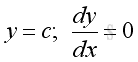Proof: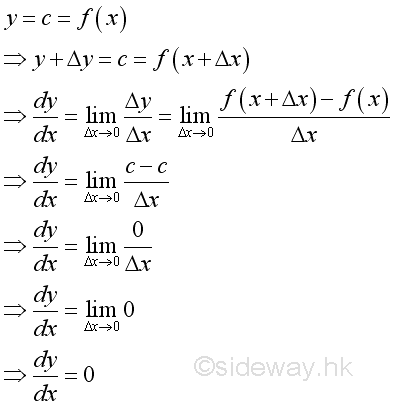2. Derivative of Linear Function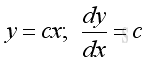Proof: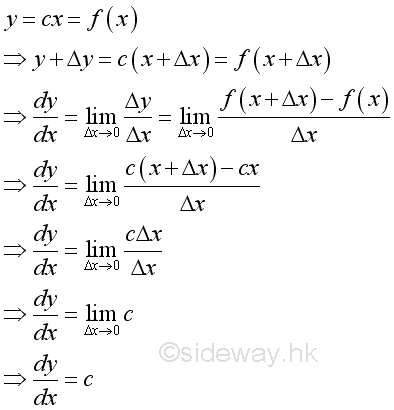3. Derivative of Power Function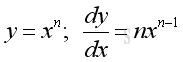Proof when n is positive: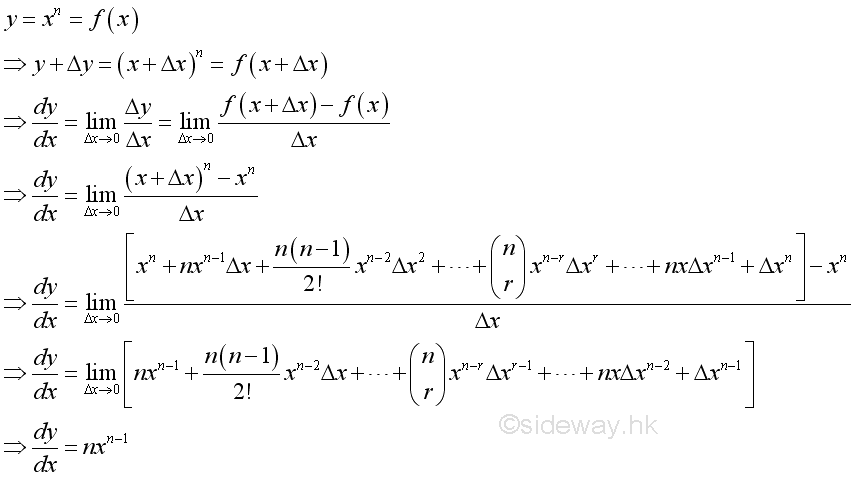Proof when n is zero and x not equal to zero: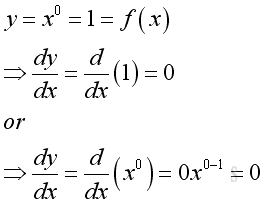n can be any real number and proof will be included in the later part of rules.

4. Rule of Constant Multiple Function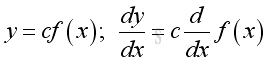Proof: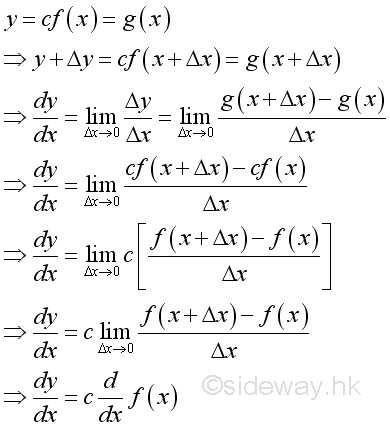5. Rule of Sum of Functions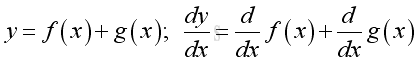Proof: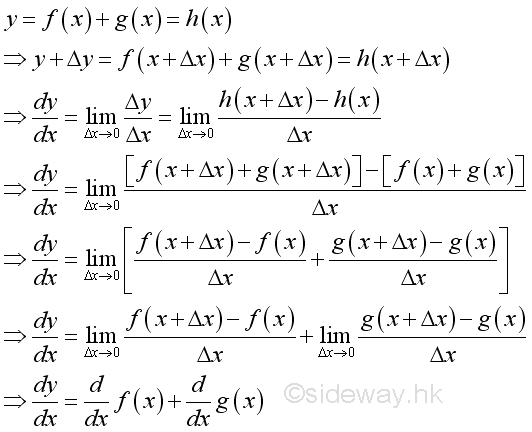The sum of functions can be extended to the sum of any functions by repeating the sum rule.

6. Rule of Difference of Functions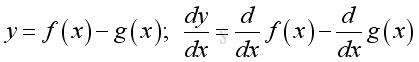Proof if both functions are differentiable: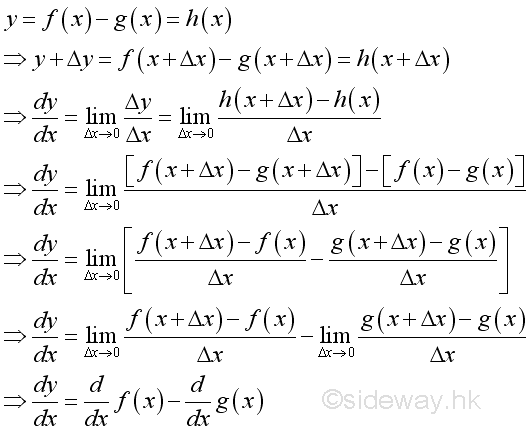The difference of functions can be extended to the difference of any functions by repeating the difference rule. And the different rule can be obtained by applying the constant multiple rule to the sum rule.

7. Rule of Product of Functions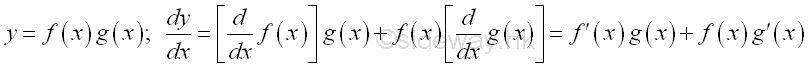Proof if both functions are differentiable: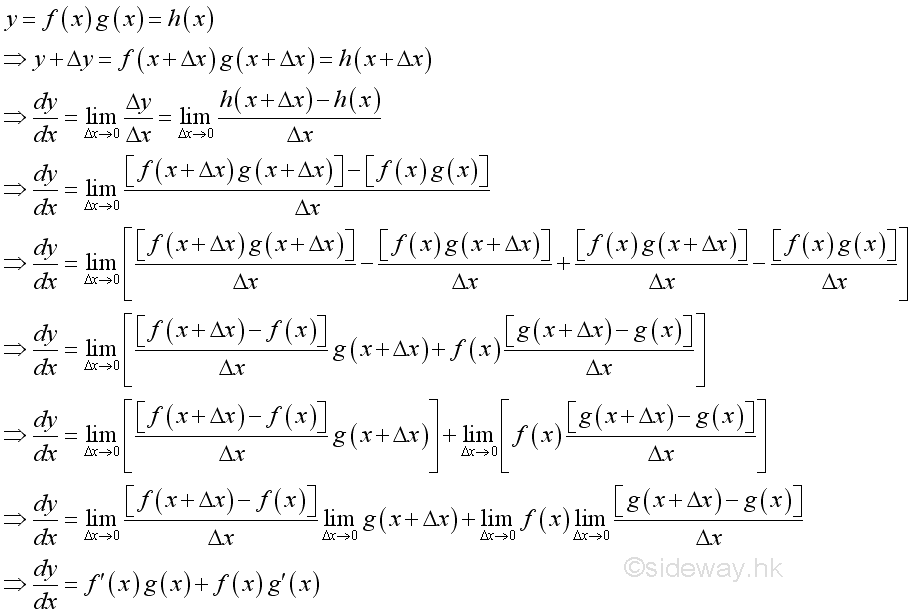The proof is completed by subtracting and adding an addition expression in the numerator. Since both functions f and g are differentiable, they are continuous and f(x)=f(x+Δx) and g(x)=g(x+Δx) when Δx approaching zero. The product rule can also be extend to any number of functions.

8. Rule of Quotient of Functions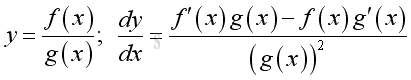Proof if both functions are differentiable: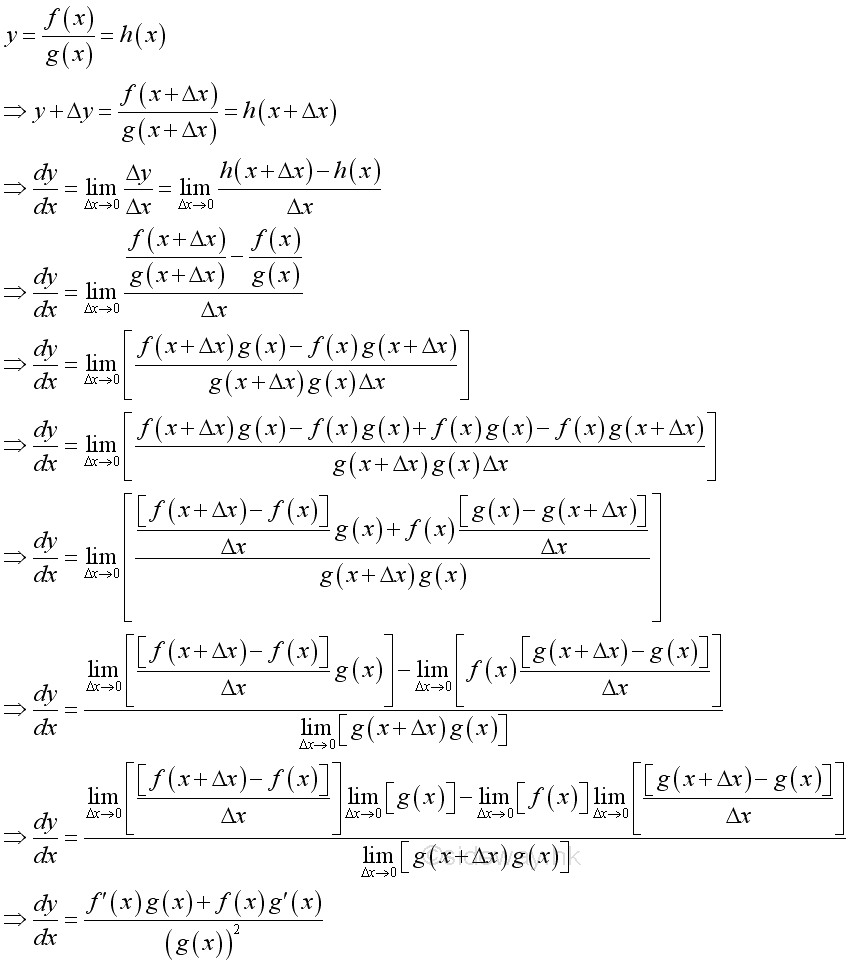The proof is completed by subtracting and adding an addition expression in the numerator. Since function g is differentiable, it is continuous and g(x)=g(x+Δx) when Δx approaching zero.

Proof for the power function when n is negative: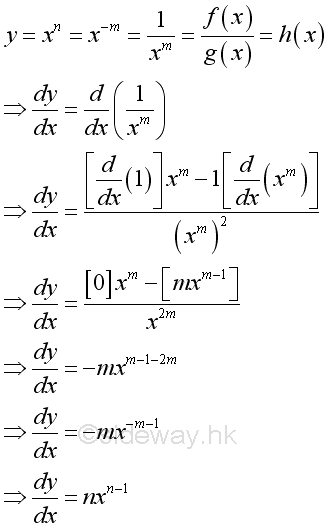9. Rule of Composite Function (The chain rule):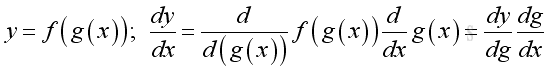Proof if both functions are differentiable:

For g(x)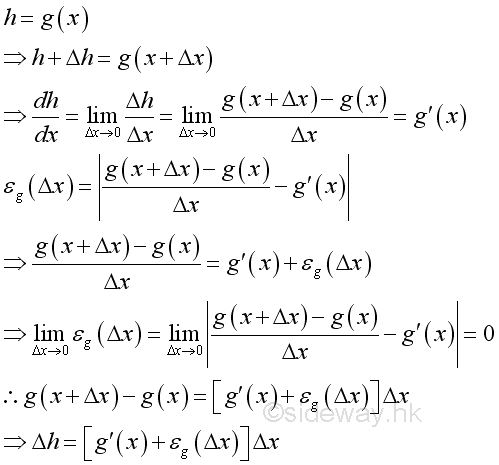For f(g(x)):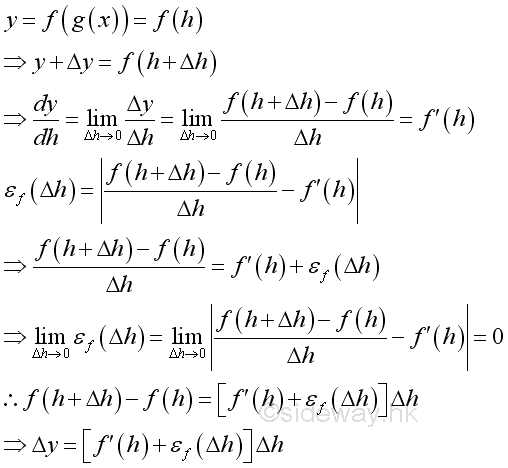Therefore: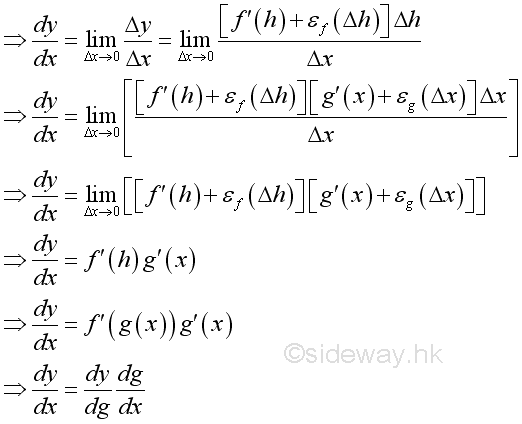Since function g is differentiable, it is continuous. When Δx approaching zero, εg equals zero and Δh is approaching zero. And therefore εf equals zero as Δx approaching zero also. The rule of composite function can be extended to any number of composite function and the derivative can be expressed in form of a chain.

10. Rule of Inverse Function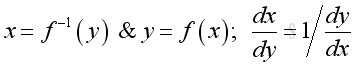Proof if both functions are differentiable and x is the inverese function of y: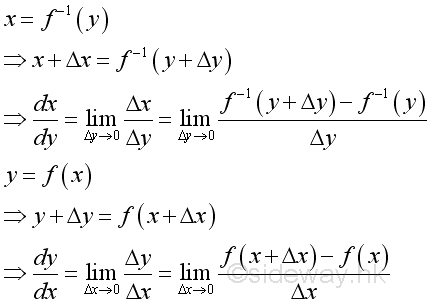Since x=f-1(y) if and only if y=f(x):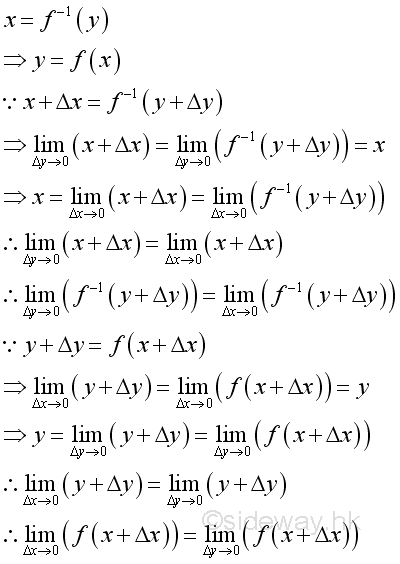Therefore: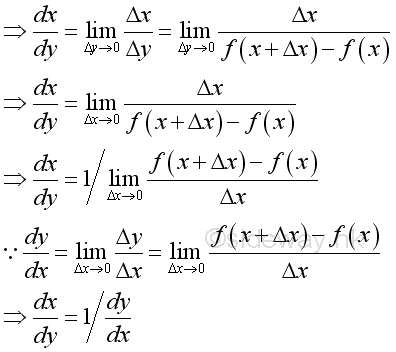Proof for the power function when n is rational number: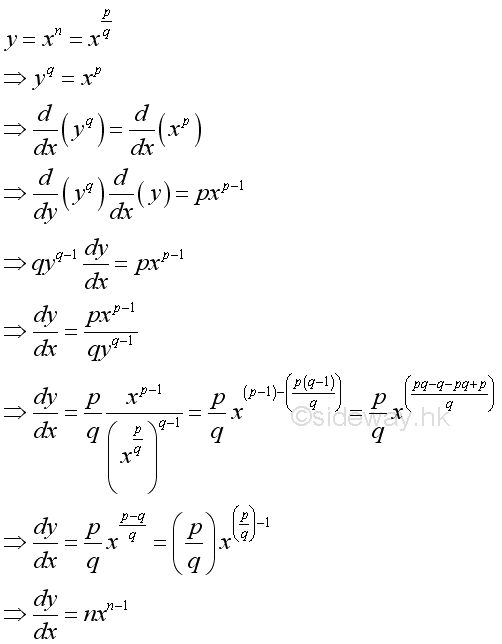ID: 110900005 Last Updated: 10/30/2011 Revision: 2 Ref:References

1. S. James, 1999, Calculus
2. B. Joseph, 1978, University Mathematics: A Textbook for Students of Science &amp; EngineeringHome 5

Management

HBR 3

Information

Recreation

Culture

Chinese 1097

English 339

Computer

Hardware 224

Software

Application 213

Latex 52

Manim 204

KB 1

Numeric 19

Programming

Web 289

Unicode 504

HTML 66

CSS 65

SVG 46

ASP.NET 270

OS 429

Python 72

Knowledge

Mathematics

Algebra 84

Geometry 33

Calculus 67

Engineering

Mechanical

Rigid Bodies

Statics 92

Dynamics 37

Control

Natural Sciences

Electric 27